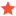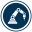• 0 Vote(s) - 0 Average
• 1
• 2
• 3
• 4
• 5
 Exporting joints position of a 6 axis robotYohan LemarchandelMemberPosts: 27 Threads: 14 Joined: May 2021 Reputation: 0 06-13-2022, 10:30 AM Hello, I'm using the JointsSpeedAccel.py Macro to export joints positions of a 6 axis robot. I would like to know what correspond to the value of the position of each axis exported ? Are there polar coordinates ? I thought that macro export joints position in cartesian coordinates with euler angles. Thanks, Yohan Attached FilesNouvelle Station (1).rdk (Size: 416 KB / Downloads: 76) Find ReplySamModeratorPosts: 244 Threads: 2 Joined: Jun 2021 Reputation: 14 06-13-2022, 12:52 PM Hi, The script exports the joint values of the robot, in degrees. You can modify the script to add the Cartesian values. Code:```# This script generates a chart of the simulated joints calculating joint speeds and accelerations # Tip: Use the script JointsPlayback.py to move along the recorded joints from robolink import *    # API to communicate with RoboDK from robodk import *      # basic matrix operations from time import gmtime, strftime, time # Simulation ratio: lower is more accurate SimulationSpeed = 0.05 # A program that takes 5 seconds in real time, will be SimulationSpeed times faster in simulation (or slower if < 1) # Sampling time: how often we want a new entry SampleTime = 0.05 # To get better results: # Select tools-Options-Motion # Set Maximum path step (mm) to 0.01 # Set Maximum path step (deg) to 0.01 # Start RoboDK API RDK = Robolink() t_ratio = 1/SimulationSpeed RDK.setSimulationSpeed(SimulationSpeed) # Ask the user to select a robot arm (mechanisms are ignored) prog = RDK.ItemUserPick('Select a program',ITEM_TYPE_PROGRAM) if not prog.Valid():    raise Exception("Robot is not available") robot = prog.getLink(ITEM_TYPE_ROBOT) ndofs = len(robot.Joints().list()) # Generate a file in the same folder where we have the RDK file file_path = RDK.getParam('PATH_OPENSTATION') + '/joints-' + prog.Name() + '.csv' def joints_changed(j1, j2, tolerance=0.0001):    """Returns True if joints 1 and joints 2 are different"""    if j1 is None or j2 is None:        return True            for j1,j2 in zip(j1,j2):        if abs(j1-j2) > tolerance:            return True                return False def diff(j1, j2, dt, dofs):    """Returns True if joints 1 and joints 2 are different"""    if j2 is None or dt <= 0:        return *dofs        res = []    for j1,j2 in zip(j1,j2):        res.append((j1-j2)/dt)        return res # Infinite loop to record robot joints print("Recording robot joints to file: " + file_path) with open(file_path,'w') as fid:    joints_header = ",".join(["Joint " + str(i+1) for i in range(ndofs)])    speeds_header = ",".join(["Speed " + str(i+1) for i in range(ndofs)])    accel_header = ",".join(["Accel " + str(i+1) for i in range(ndofs)])    xyzwpr_header = ",".join(["X", "Y", "Z", "W", "P", "R"])    fid.write("Time (s)," + joints_header + ",,Time (s)," + speeds_header + ",,Time (s)," + accel_header + ",,Time (s)," + xyzwpr_header)    fid.write("\n")    joints_last = None    speeds_last = None    t_last = None    t_start = None        RDK.Render(True)    prog.RunProgram()    while prog.Busy():        #t_now = time() # Estimate using t_ratio        #t_now = float(RDK.Command("SimulationTime"))*0.001                t_now = float(RDK.Command("TrajectoryTime")) # RoboDK's internal clock for motion simulation        joints = robot.Joints().list()        if joints_changed(joints, joints_last):                                    if t_last is None:                t_last = t_now                t_start = t_now            # Calculate timings            #t_sim = t_ratio * (t_now - t_start) # not needed if we use RoboDK's SimulationTime            #t_delta = t_ratio * (t_now - t_last) # not needed if we use RoboDK's SimulationTime            t_sim = t_now - t_start            t_delta = t_now - t_last            if t_delta < SampleTime:                # Minimum 5 ms time for good accuracy                continue            # Calculate speeds            speeds = diff(joints, joints_last, t_delta, ndofs)                        # Calcualte accelerations            accels = diff(speeds, speeds_last, t_delta, ndofs)                        # Calculate xyz            xyzwpr = pose_2_xyzrpw(robot.Pose())            print('Time +S: %.3f s' % t_sim)            joints_str = ",".join(["%.6f" % x for x in joints])            speeds_str = ",".join(["%.6f" % x for x in speeds])            accels_str = ",".join(["%.6f" % x for x in accels])            xyzwpr_str = ",".join(["%.6f" % x for x in xyzwpr])                        time_str = ("%.6f," % t_sim)            fid.write(time_str + joints_str + ",," + time_str + speeds_str + ",," + time_str + accels_str + ",," + time_str + xyzwpr_str)            fid.write("\n")            t_last = t_now            joints_last = joints            speeds_last = speeds            #pause(0.005)            ``` Please read the Forum Guidelines before posting! Find useful information about RoboDK by visiting our Online Documentation. Find ReplyAlbertModeratorPosts: 1,921 Threads: 1 Joined: Apr 2018 Reputation: 101 06-13-2022, 01:29 PM This script only exports joint values, not Cartesian. You can calculate the Cartesian position of your robot effector using SolveFK. Find Reply

 Users browsing this thread: 1 Guest(s)